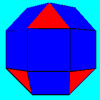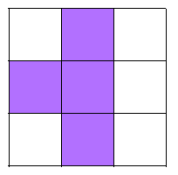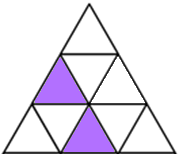#### You may also like### Eight Dominoes

Using the 8 dominoes make a square where each of the columns and rows adds up to 8### Rhombicubocts

Each of these solids is made up with 3 squares and a triangle around each vertex. Each has a total of 18 square faces and 8 faces that are equilateral triangles. How many faces, edges and vertices does each solid have?### Prime Magic

Place the numbers 1, 2, 3,..., 9 one on each square of a 3 by 3 grid so that all the rows and columns add up to a prime number. How many different solutions can you find?

##### Age 11 to 14Challenge Level

Shady Symmetry printable sheet - problem
Printable isometric grid template

Charlie created a symmetrical pattern by shading in four squares on a 3 by 3 square grid:Alison created a symmetrical pattern by shading in two triangles on a 3 by 3 isometric grid:Choose whether you would like to work on square grids or isometric grids.

How many different symmetrical patterns can you make?

Here are some questions you might like to consider:
• How many different patterns can you make if you are only allowed to shade in one... two... three... four cells?
• How does the number of patterns with 6 cells shaded relate to the number with 3 cells shaded?
• Can you make patterns with exactly one... two... three... four lines of symmetry?
• Can you make patterns with rotational symmetry AND lines of symmetry?
• Can you make patterns with rotational symmetry but NO lines of symmetry?
• Can you make patterns using more than one colour?